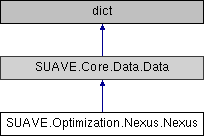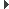SUAVE  2.5.2 An Aerospace Vehicle Environment for Designing Future AircraftSUAVE.Optimization.Nexus.Nexus Class Reference
Inheritance diagram for SUAVE.Optimization.Nexus.Nexus:## Public Member Functions

def __defaults__ (self)

def evaluate (self, x=None)

def objective (self, x=None)

def inequality_constraint (self, x=None)

def equality_constraint (self, x=None)

def all_constraints (self, x=None)

def unpack_inputs (self, x=None)

def constraints_individual (self, x=None)

def finite_difference (self, x, diff_interval=1e-8)

def translate (self, x=None)Public Member Functions inherited from SUAVE.Core.Data.Data
def __getattribute__ (self, k)

def __setattr__ (self, k, v)

def __delattr__ (self, k)

def __new__ (cls, *args, **kwarg)

def typestring (self)

def dataname (self)

def __str__ (self, indent='')

def __init__ (self, *args, **kwarg)

def __iter__ (self)

def itervalues (self)

def values (self)

def update (self, other)

def append_or_update (self, other)

def get_bases (self)

def append (self, value, key=None)

def deep_set (self, keys, val)

def deep_get (self, keys)

def pack_array (self, output='vector')

def unpack_array (self, M)

def do_recursive (self, method, other=None, default=None)

## Public Attributes

vehicle_configurations

analyses

missions

procedure

results

summary

optimization_problem

fidelity_level

last_inputs

last_fidelity

evaluation_count

force_evaluate

hard_bounded_inputs

## Detailed Description

```noun (plural same or nexuses)
-a connection or series of connections linking two or more things
-a connected group or series: a nexus of ideas.
-the central and most important point or place

This is the class that makes optimization possible. We put all the data and functions together to make

Assumptions:
You like SUAVE

Source:
Oxford English Dictionary
```

## ◆ __defaults__()

 def SUAVE.Optimization.Nexus.Nexus.__defaults__ ( self )
```This sets the default values.

Assumptions:
None

Source:
N/A

Inputs:
None

Outputs:
None

Properties Used:
None
```

Reimplemented from SUAVE.Core.Data.Data.

## ◆ all_constraints()

 def SUAVE.Optimization.Nexus.Nexus.all_constraints ( self, x = `None` )
```Returns both the inequality and equality constraint values for your function

Assumptions:
N/A

Source:
N/A

Inputs:
x                  [vector]

Outputs:
scaled_constraints [vector]

Properties Used:
None
```

## ◆ constraints_individual()

 def SUAVE.Optimization.Nexus.Nexus.constraints_individual ( self, x = `None` )
```Put's the values of the problem in the right place.

Assumptions:
N/A

Source:
N/A

Inputs:
x                  [vector]

Outputs:
None

Properties Used:
None
```

## ◆ equality_constraint()

 def SUAVE.Optimization.Nexus.Nexus.equality_constraint ( self, x = `None` )
```Retrieve the equality constraint values for your function

Assumptions:
N/A

Source:
N/A

Inputs:
x                  [vector]

Outputs:
scaled_constraints [vector]

Properties Used:
None
```

## ◆ evaluate()

 def SUAVE.Optimization.Nexus.Nexus.evaluate ( self, x = `None` )
```This function runs the problem you setup in SUAVE.
If the last time you ran this the inputs were the same, a cache is used.

Assumptions:
None

Source:
N/A

Inputs:
x       [vector]

Outputs:
None

Properties Used:
None
```

## ◆ finite_difference()

 def SUAVE.Optimization.Nexus.Nexus.finite_difference ( self, x, diff_interval = `1e-8` )
```Finite difference gradients and jacobians of the problem.

Assumptions:
N/A

Source:
N/A

Inputs:
x                  [vector]
diff_interval      [float]

Outputs:
jac_con            [array]

Properties Used:
None
```

## ◆ inequality_constraint()

 def SUAVE.Optimization.Nexus.Nexus.inequality_constraint ( self, x = `None` )
```Retrieve the inequality constraint values for your function

Assumptions:
N/A

Source:
N/A

Inputs:
x                  [vector]

Outputs:
scaled_constraints [vector]

Properties Used:
None```

## ◆ objective()

 def SUAVE.Optimization.Nexus.Nexus.objective ( self, x = `None` )
```Retrieve the objective value for your function

Assumptions:
N/A

Source:
N/A

Inputs:
x       [vector]

Outputs:
scaled_objective [float]

Properties Used:
None
```

## ◆ translate()

 def SUAVE.Optimization.Nexus.Nexus.translate ( self, x = `None` )
```Make a pretty table view of the problem with objective and constraints at the current inputs

Assumptions:
N/A

Source:
N/A

Inputs:
x                  [vector]

Outputs:
inpu               [array]
const_table        [array]

Properties Used:
None
```

## ◆ unpack_inputs()

 def SUAVE.Optimization.Nexus.Nexus.unpack_inputs ( self, x = `None` )
```Put's the values of the problem in the right place.

Assumptions:
N/A

Source:
N/A

Inputs:
x                  [vector]

Outputs:
None

Properties Used:
None
```

The documentation for this class was generated from the following file:
• /Users/emiliobotero/Dropbox/SUAVE/SUAVE/trunk/SUAVE/Optimization/Nexus.py